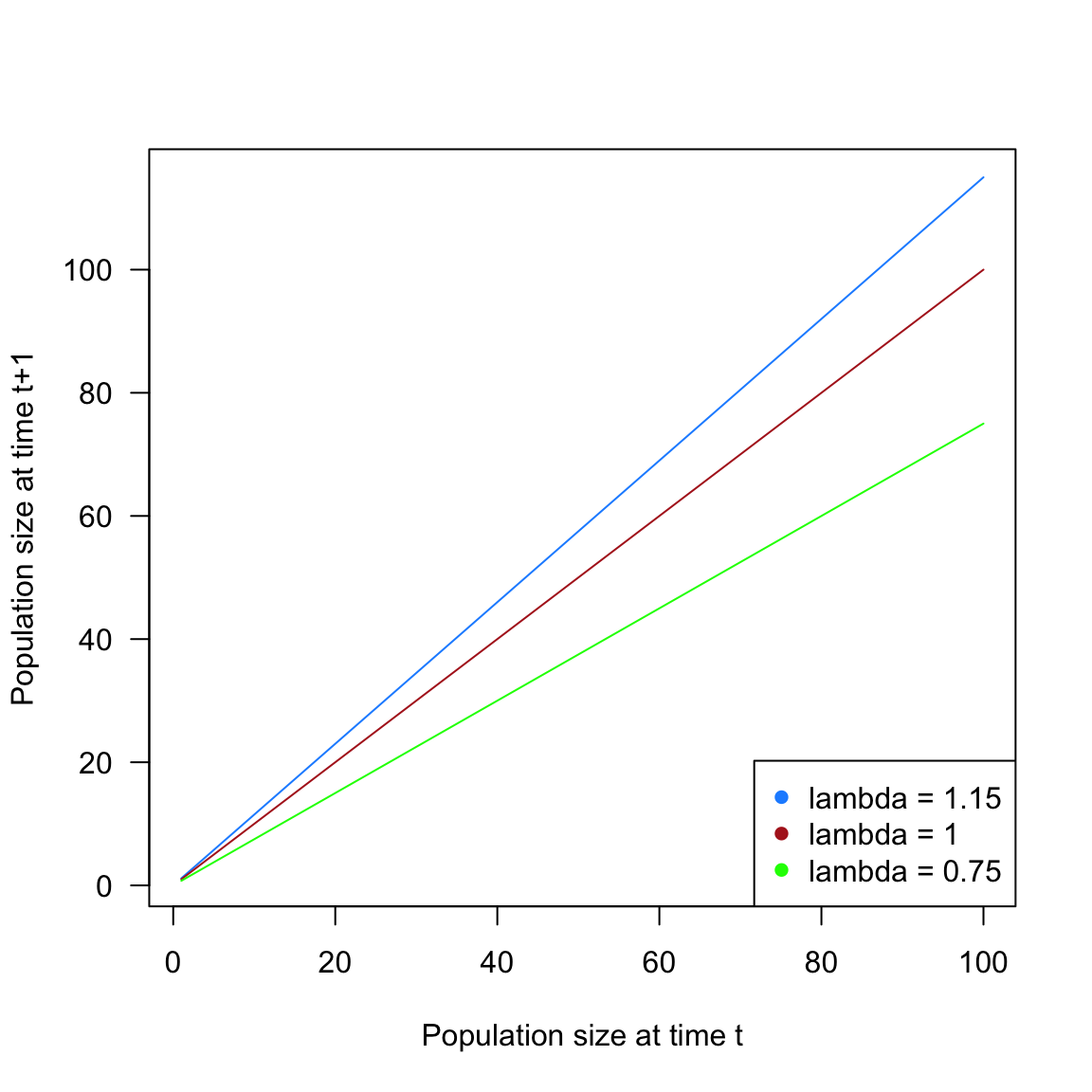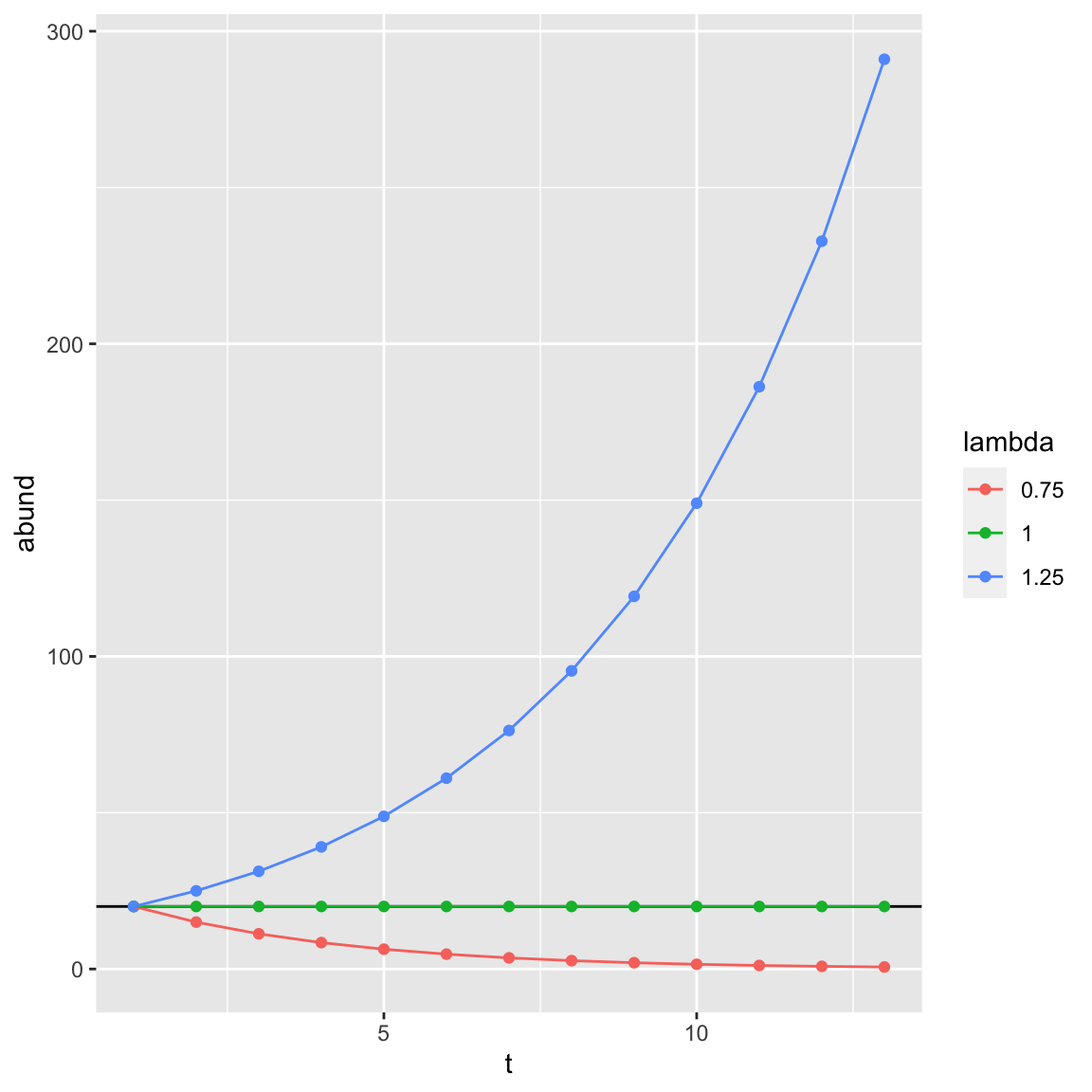((use this lecture to discuss the concepts of stable and unstable equilibrium))

## Exponential growth

``````expoGrowth <- function(n, lambda){
n * lambda
}

n0 <- 1:100

# effect of growth rate
plot(x = n0, y = expoGrowth(n0, lambda = 1.15), type = 'l', las = 1,
xlab = 'Population size at time t',
ylab = 'Population size at time t+1', col = 'dodgerblue'
)
lines(n0, expoGrowth(n0, lambda = 1), col = 'firebrick')
lines(n0, expoGrowth(n0, lambda = 0.75), col = 'green')

legend('bottomright',
paste(expression(lambda), c('= 1.15', '= 1', '= 0.75')),
pch = 16, col = c('dodgerblue', 'firebrick', 'green')
)````````````# exponential growth
expoDynamics <- function(n, lambda, steps = 100){
ret <- c()
ret <- n
for(i in 1:steps){
ret[i+1] <- expoGrowth(ret[i], lambda)
}
return(ret)
}``````
``````library(ggplot2)
t <- 12
n0 <- 20
d <- data.frame(t = rep(1:(t + 1), 3),
lambda = as.factor(rep(c(0.75, 1, 1.25), each = t + 1)),
abund = c(
expoDynamics(n0, lambda = 0.75, steps = t),
expoDynamics(n0, lambda = 1, steps = t),
expoDynamics(n0, lambda = 1.25, steps = t)
))

ggplot(data = d, aes(x = t, y = abund, color = lambda, group = lambda)) +
geom_hline(yintercept = n0) +
geom_point() +
geom_line()``````## Logistic growth

``````n0 <- 1:100
k <- 20
r <- 0.5

logisticGrowth <- function(n, r, k) {
n * exp(r * (1 - (n / k)))
}

colz <- c(grey(0.1, 0.9), 'dodgerblue', 'firebrick', 'forestgreen')
#effect of growth rate
plot(n0, logisticGrowth(n = n0, r = 2.7, k = 20),
type='l', las=1,
xlab='Population size at time t',
ylab='Population size at time t+1',
col="red")
lines(n0, logisticGrowth(n = n0, r = 1.5, k = 20), col = "orange")
abline(a = 0, b = 1, col = "blue")
abline(a = 40, b = -1, col = "blue")
legend('topright', bty='n', c('r=2.7', 'r=1.5'),
pch = 16, col = c("red", "orange"))``````